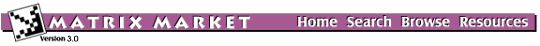BCSSTK31: BCS Structural Engineering Matrices (large eigenvalue problems)Statics module of an automobile component

from set BCSSTRUC5, from the Harwell-Boeing Collection

Stiffness matrix

Help: My browser can't read the compressed data files. What now?

Visualizations

Click on image to get an enlarged version. Click on label to get an explanation.

Matrix Statistics

* * * * * * * * * * * * * * * * * * * * * * * * * * * * * * * * * * * * * *
*                           Matrix Statistics                             *
*              Generated by Yousef Saad's SPARSKIT Library                *
* * * * * * * * * * * * * * * * * * * * * * * * * * * * * * * * * * * * * *
* * * * * * * * * * * * * * * * * * * * * * * * * * * * * * * * * * * * * *
* TIFFNESS MATRIX FOR AUTOMOBILE COMPONENT (MSC NASTRAN) - PATTERN ONLY   *
*                    Key = BCSSTK31 , Type = PSA                          *
* No values provided - Information on pattern only                        *
* * * * * * * * * * * * * * * * * * * * * * * * * * * * * * * * * * * * * *
*  Dimension N                                      =      35588  *
*  Number of nonzero elements                       =     608502  *
*  Average number of nonzero elements/Column        =    17.0985  *
*  Standard deviation for above average             =     9.2808  *
*  Nonzero elements in strict lower part            =     572914  *
*  Nonzero elements in strict upper part            =     572914  *
*  Nonzero elements in main diagonal                =      35588  *
*  Weight of longest column                         =        197  *
*  Weight of shortest column                        =          2  *
*  Matching elements in symmetry                    =          0  *
*  Relative Symmetry Match (symmetry=1)             = NaN         *
*  Average distance of a(i,j)  from diag.           =  0.379E+03  *
*  Standard deviation for above average             =  0.349E+03  *
*-----------------------------------------------------------------*
*  Lower bandwidth  (max: i-j, a(i,j) .ne. 0)       =          0  *
*  Upper bandwidth  (max: j-i, a(i,j) .ne. 0)       =       1668  *
*  Maximum Bandwidth                                =       1669  *
*  Average Bandwidth                                =  0.620E+03  *
*  Number of nonzeros in skyline storage            =   23198053  *
*  90% of matrix is in the band of width            =       1731  *
*  80% of matrix is in the band of width            =       1465  *
*  The total number of nonvoid diagonals is         =       1550  *
*  The  9 most important diagonals are (offsets)    :             *
*     0     1     5     2     4     6     3     7     8           *
*  The accumulated percentages they represent are   :             *
*   5.8   9.7  13.0  16.0  18.7  21.1  23.3  25.0  26.2           *
*-----------------------------------------------------------------*
*  The matrix does not have a block structure                     *
*-----------------------------------------------------------------*

Set Information

Set BCSSTRUC5
Source: Boeing Computer Services
Discipline:Structural engineering
Accession:Spring 1986

The Matrix Market is a service of the Mathematical and Computational Sciences Division / Information Technology Laboratory / National Institute of Standards and Technology.

[ Home ] [ Search ] [ Browse ] [ Resources ]